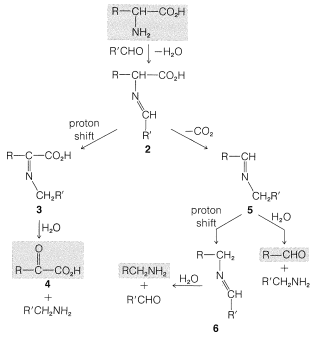# 25.5: Reactions of Amino Acids

$$\newcommand{\vecs}{\overset { \rightharpoonup} {\mathbf{#1}} }$$ $$\newcommand{\vecd}{\overset{-\!-\!\rightharpoonup}{\vphantom{a}\smash {#1}}}$$$$\newcommand{\id}{\mathrm{id}}$$ $$\newcommand{\Span}{\mathrm{span}}$$ $$\newcommand{\kernel}{\mathrm{null}\,}$$ $$\newcommand{\range}{\mathrm{range}\,}$$ $$\newcommand{\RealPart}{\mathrm{Re}}$$ $$\newcommand{\ImaginaryPart}{\mathrm{Im}}$$ $$\newcommand{\Argument}{\mathrm{Arg}}$$ $$\newcommand{\norm}{\| #1 \|}$$ $$\newcommand{\inner}{\langle #1, #2 \rangle}$$ $$\newcommand{\Span}{\mathrm{span}}$$ $$\newcommand{\id}{\mathrm{id}}$$ $$\newcommand{\Span}{\mathrm{span}}$$ $$\newcommand{\kernel}{\mathrm{null}\,}$$ $$\newcommand{\range}{\mathrm{range}\,}$$ $$\newcommand{\RealPart}{\mathrm{Re}}$$ $$\newcommand{\ImaginaryPart}{\mathrm{Im}}$$ $$\newcommand{\Argument}{\mathrm{Arg}}$$ $$\newcommand{\norm}{\| #1 \|}$$ $$\newcommand{\inner}{\langle #1, #2 \rangle}$$ $$\newcommand{\Span}{\mathrm{span}}$$

## Ester and Amide Formation

To some degree the reactions of amino acids are typical of isolated carboxylic acid and amine functions. Thus the carboxyl function can be esterified with an excess of an alcohol under acidic conditions, and the amine function can be acylated with acid chlorides or anhydrides under basic conditions:The products, however, are not indefinitely stable because the functional groups can, and eventually will, react with each other. For example, in the acylation of glycine with ethanoic anhydride, the first-formed product may cyclize to the "azlactone" if the reaction is prolonged or excess anhydride is used:Esters of amino acids also cyclize, but they do so intermolecularly to give "diketopiperazines". These compounds are cyclic amides:## Nitrous Acid Reaction

The amine function of $$\alpha$$-amino acids and esters reacts with nitrous acid in a manner similar to that described for primary amines (Section 23-10A). The diazonium ion intermediate loses molecular nitrogen in the case of the acid, but the diazonium ester loses a proton and forms a relatively stable diazo compound known as ethyl diazoethanoate:This diazo ester is formed because loss of $$\ce{N_2}$$ from the diazonium ion results in formation of a quite unfavorable carbocation.

## Amino Acids with Aldehydes

$$\alpha$$-Amino acids react with aldehydes to form decarboxylation and/or deamination products. The reaction sequence is shown in Figure 25-5 and closely resembles the ninhydrin reaction (Section 25-4A). In the first step the amine condenses with the aldehyde to give an imine or Schiff base, $$2$$. What happens next depends on the relative rates of proton shift and decarboxylation of $$2$$. Proton shift produces a rearranged imine, $$3$$, which can hydrolyze to the keto acid $$4$$. The keto acid is a deamination product. Alternatively, decarboxylation can occur (see Section 18-4) and the resulting imine, $$5$$, can either hydrolyze or rearrange by a proton shift to a new imine, $$6$$. Hydrolysis of $$5$$ or $$6$$ gives an aldehyde and an amine.Figure 25-5: Reactions of $$\alpha$$-amino acids with an aldehyde, $$\ce{R'CHO}$$. The products are the result of decarboxylation and/or deamination; the fraction of the products formed by each route is determined by the ratio of the rate of proton shift to the rate of decarboxylation of $$2$$.

There is an important biochemical counterpart of the deamination reaction that utilizes pyridoxal phosphate, $$7$$, as the aldehyde. Each step in the sequence is catalyzed by a specific enzyme. The $$\alpha$$-amino group of the amino acid combines with $$7$$ and is converted to a keto acid. The resulting pyridoxamine then reacts to form an imine with a different $$\alpha$$-keto acid, resulting in formation of a new $$\alpha$$-amino acid and regenerating $$7$$. The overall process is shown in Equation 25-6 and is called transamination. It is a key part of the process whereby amino acids are metabolized.$$\tag{25-6}$$

The biochemical process occurs with complete preservation of the $$L$$ configuration at the $$\alpha$$ carbon. The same reactions can be carried out nonenzymatically using pyridoxal phosphate, but they are nonstereospecific, require metal ions as a catalyst, and give mixtures of products.